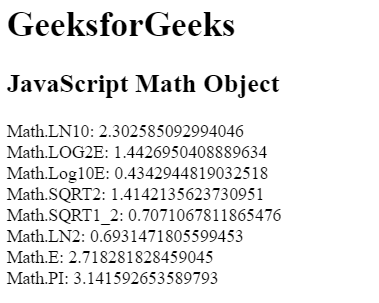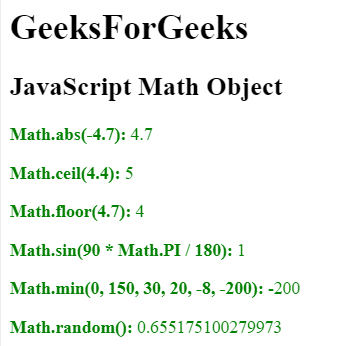# JavaScript | Math Object

• Difficulty Level : Easy
• Last Updated : 01 Mar, 2019

The Math object is used to perform mathematical operations on numbers. There are many math object properties which are listed below:

PropertyDescription
Math.EEuler’s number
Math.PIPI
Math.SQRT2The square root of 2
Math.SQRT1_2The square root of 1/2
Math.LN2The natural logarithm of 2
Math.LN10The natural logarithm of 10
Math.LOG2EBase 2 logarithm of E
Math.LOG10EBase 10 logarithm of E

Math objects: There are many math objects exists in JavaScript which are listed below:

Hey geek! The constant emerging technologies in the world of web development always keeps the excitement for this subject through the roof. But before you tackle the big projects, we suggest you start by learning the basics. Kickstart your web development journey by learning JS concepts with our JavaScript Course. Now at it's lowest price ever!

PropertyDescription
abs(x)Absolute value of x
atan(x)Arctangent of x, a numeric value between -PI/2 and PI/2 radian
atan2(y, x)Arctangent of the quotient of its arguments
ceil(x)Value of x rounded up to the nearest integer
cos(x)Cosine of x (x in radians)
exp()Value of E^x
floor()Value of x rounded below to the nearest integer
log()Natural logarithm (base E) of x
max(a, b, …)Highest value
min(a, b, …)Lowest value
pow(x, y)Value of x to power of y
random()Random number between 0 and 1
round(x)Value of x rounded to the nearest integer
sin(x)Sine of x (x in radians)
sqrt(x)Square root of x
tan(x)Tangent of angle

Example 1: This example use math object properties to return their values.

 ` ``<``html``> `` ` `<``head``> ``    ``<``title``> ``        ``JavaScript Math Object``    `` `` ``     ` `<``body``> `` ` `    ``<``h1``>GeeksforGeeks ``         ` `    ``<``h2``>JavaScript Math Object `` ` `    ``<``p` `id``=``"GFG"``>``     ` `    ```    `    ``<``script``> ``        ``document.getElementById("GFG").innerHTML = ``            ``"Math.LN10: " + Math.LN10 + "<``br``>" +``            ``"Math.LOG2E: " + Math.LOG2E + "<``br``>" +``            ``"Math.Log10E: " + Math.LOG10E + "<``br``>" +``            ``"Math.SQRT2: " + Math.SQRT2 + "<``br``>" +``            ``"Math.SQRT1_2: " + Math.SQRT1_2 + "<``br``>" +``            ``"Math.LN2: " + Math.LN2 + "<``br``>" +``            ``"Math.E: " + Math.E + "<``br``>" +``            ``"Math.PI: " + Math.PI;``    `` `` `` ` `                    `

Output:Example 2: Math object methods are used in this example.

 ` ``<``html``> `` ` `<``head``> ``    ``<``title``> ``        ``JavaScript Math Object``    `` `` ``     ` `<``body``> `` ` `    ``<``h1``>GeeksForGeeks ``         ` `    ``<``h2``>JavaScript Math Object `` ` `    ``<``p` `id``=``"GFG"` `style` `= ``"color:green;"``>``     ` `    ```    `    ``<``script``> ``        ``document.getElementById("GFG").innerHTML = ``        ``"<``p``><``b``>Math.abs(-4.7): " + Math.abs(-4.7) + "" +``        ``"<``p``><``b``>Math.ceil(4.4): " + Math.ceil(4.4) + "" +``        ``"<``p``><``b``>Math.floor(4.7): " + Math.floor(4.7) + "" +``        ``"<``p``><``b``>Math.sin(90 * Math.PI / 180): " + ``                ``Math.sin(90 * Math.PI / 180) + "" +``        ``"<``p``><``b``>Math.min(0, 150, 30, 20, -8, -200): " +``                ``Math.min(0, 150, 30, 20, -8, -200) + "" +``        ``"<``p``><``b``>Math.random(): " + Math.random() + "";``    `` `` `` ` `                    `

Output:My Personal Notes arrow_drop_up